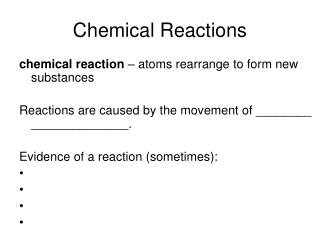# Chemical Reactions - PowerPoint PPT PresentationDownload PresentationChemical Reactions

Chemical ReactionsDownload Presentation## Chemical Reactions

- - - - - - - - - - - - - - - - - - - - - - - - - - - E N D - - - - - - - - - - - - - - - - - - - - - - - - - - -
##### Presentation Transcript

1. Chemical Reactions chemical reaction – atoms rearrange to form new substances Reactions are caused by the movement of ________ ______________. Evidence of a reaction (sometimes):

2. Chemical Equations reactants – chemicals that you start with products – chemicals formed during reaction A chemical equation looks like this: reactants  products which literally means “reactants react to form products” or “reactants yield products”

3. Symbols in Chemical Equations

4. Symbols in Chemical Equations Zn(s) + H2O(l) ZnO(s) + H2(g) Na2CO3(aq) + Ca(OH)2(aq)  NaOH(aq) + CaCO3(s) CaCO3(s)  CaO(s) + CO2(g)

5. Writing Chemical Equations Solid sodium oxide reacts with liquid water to form a solution of sodium hydroxide. Hydrogen chloride solution and zinc metal form zinc chloride (ZnCl2) solution and hydrogen gas (H2).

6. Chemical Equations coefficients 2H2 + O2 2H2O subscript – number of each atom in a compound - no subscript means there is 1 atom coefficient – number of each compound - no coefficient means there is 1 compound subscripts

7. Writing Chemical Reactions To get the total number of atoms, multiply the coefficient by the subscript. 4Fe + 3O2 2Fe2O3 4Fe means that there are 4 x 1 = 4 iron atoms on the reactant side 3O2 means that there are 3 x 2 = 6 oxygen atoms on the reactant side 2Fe2O3 means that there are 2 x 2 = 4 iron atoms and 2 x 3 = 6 oxygen atoms on the product side

8. Balancing Chemical Equations Law of Conservation of Mass states that what you put in is what you get out. In chemical reactions, that means that if you have 30 iron atoms on the reactant side, then there must be 30 iron atoms on the product side. They may be in different compounds, but the total number of atoms must be the same on each side.

9. Balancing Chemical Equations To balance an equation, change the coefficients to make the number of atoms of each element equal. DO NOT CHANGE THE SUBSCRIPTS!! Na + Cl2 NaCl TiCl4 + H2O  TiO2 + HCl Al + F2  Al2F3

10. Balancing Chemical Reactions C3H8 + O2 CO2 + H2O Sb + O2 Sb4O6 N2O5 + H2O  HNO3

11. Balancing with Polyatomic Ions Still have to count the total number of each atom on each side. Multiply subscript outside parentheses by subscript inside parentheses. DO NOT CHANGE THE SUBCRIPTS!! HNO3 + Cu  Cu(NO3)2 + H2 B(CN)3 + H2  B + HCN Al(OH)3 + HCl  AlCl3 + H2O

12. Balancing with Polyatomic Ions Ca3(PO4)2 + SiO2 P4O10 + CaSiO3 Ca(ClO3)2 CaCl2 + O2 Ag2S + KCN  KAg(CN)2 + K2S

13. Reaction Types • synthesis – two or more reactants form a single product A + B  AB Fe + Cl2 FeCl3

14. Reaction Types • decomposition – single reactant breaks down into two or more products AB  A + B HgO  Hg + O2

15. Reaction Types • single-displacement A + BX  AX + B Cu + AgNO3 Cu(NO3)2 + Ag • double-displacement AX + BY  AY + BX NaOH + CuCl2  NaCl + Cu(OH)2

16. Reaction Types • combustion – oxygen reacts with another element or compound AND releases heat and light CH4 + O2 CO2 + H2O Sometimes combustion reactions are synthesis as well. Mg + O2  MgO

17. Activity Series definition – order of reactivity of elements (most reactive on top, least reactive on bottom) Element will displace element below it – reaction occurs Element will NOT displace element above it – reaction does NOT occur

18. Activity Series Predict whether or not the following reactions will occur. Na(s) + MgBr2(aq)  Mn(NO3)2(aq) + Au(s)  Hg(s) + PtO(aq) 

19. Predicting Reaction Products • If there is only one reactant, write the individual elements then skip to step 5. • If there is more than one reactant, label each atom/ion with charge. • Form resulting compounds using correct charge combinations – ignore subscripts on the reactant side. • Using the charges, put the correct subscripts on the products. • Balance the reaction.

20. Reaction Prediction Determine the products and balance the rxn. K(s) + Cl2(g)  CaF2(aq) + Li2S(s)  BaS(s)  Sr2N3(aq) + Na2O(aq) 